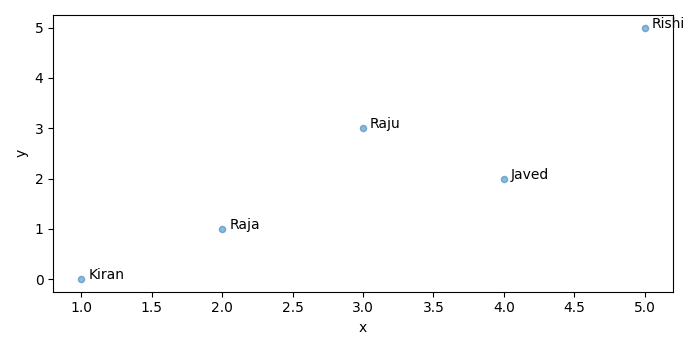# How to label bubble chart/scatter plot with column from Pandas dataframe?

To label bubble charts/scatter plot with column from Pandas dataframe, we can take the following steps −

• Set the figure size and adjust the padding between and around the subplots.
• Create a data frame, df, of two-dimensional, size-mutable, potentially heterogeneous tabular data.
• Create a scatter plot with df.
• Annotate each data point with a text.
• To display the figure, use show() method.

## Example

import pandas as pd
from matplotlib import pyplot as plt

# Set the figure size
plt.rcParams["figure.figsize"] = [7.00, 3.50]
plt.rcParams["figure.autolayout"] = True

# Create a dataframe
df = pd.DataFrame(
dict(
x=[1, 3, 2, 4, 5],
y=[0, 3, 1, 2, 5],
points=['Kiran', 'Raju', 'Raja', 'Javed', 'Rishi']
)
)

# Scatter plot
ax = df.plot.scatter(x='x', y='y', alpha=0.5)

# Annotate each data point
for i, txt in enumerate(df.points):
ax.annotate(txt, (df.x.iat[i]+0.05, df.y.iat[i]))

plt.show()

## Output

It will produce the following output